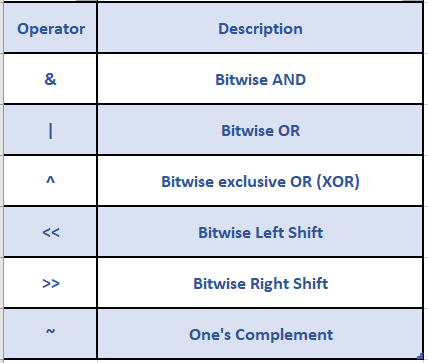In this tutorial, we are going to learn about bitwise operators in C Language.

## C Bitwise Operators:

Bitwise operators are used for manipulating data at the bit level (binary data).

Bitwise operators can be applied only to operands of integral type i.e char, int, signed, unsigned and long. C provides six bitwise operators as given below:## 1. Bitwise AND:

The format for using a Bitwise AND (&) operator is:

``operand1 & operand2``

where operand1 and operand2 should be of integral type.

It sets a bit to 1 if and only if both the corresponding bits in its operands are 1, and to 0 if the bits differ or both are 0. For example, 1010 & 1100 will result in 1000.

``````10 ⟹ 1010
&
12 ⟹ 1100
-------
1000 ⟹ 8
-------
``````

## 2. Bitwise OR:

The syntax for using a Bitwise OR (|) operator is:

``operand1 | operand2``

where operand1 and operand2 should be of integral type. It sets a bit to 1 if one or both the bits in its operands are 1, and to 0 if both the bits are 0. For example, 1010 | 1100 will result in 1110.

``````10 ⟹ 1010
|
12 ⟹ 1100
-------
1110 ⟹ 14
-------
``````

## 3. Bitwise XOR:

The syntax for using a Bitwise XOR (^) operator is:

``operand1 ^ operand2``

where operand1 and operand2 should be of integral type. It sets the bit to 1 where the corresponding bits in its operands are different, and to 0 if they are the same. For example, 1010 ^ 1100 will result in 0110.

``````10 ==> 1010
^
12 ==> 1100
------
0110  ==> 6
------``````

## 4. Bitwise Shift Operator:

### 4.1 Left shift

The syntax for using a bitwise left-shift (<<) operator is:

``operand1 << operand2``

where operand1 should be of integral type and operand2 should also be an integral number which indicates the number of positions that should be shifted to the left in operand1.

The value of number << bitstobeshifted is the number left-shifted by bitstobeshifted bit positions. This is equal to multiplying operand1 by 2bitstobeshifted.

The two examples given below show the usage of the bitwise left-shift operator (<<) on decimal 3:
Decimal 3 in binary is 11.
Hence, 11 << 1 will result in 110, i.e, 3 << 1 = 3 X 21 = 6.
Similarly, 11 << 2 will result in 1100, i.e, 3 << 2 = 3 X 22 = 12.

### 4.2 Right shift

The syntax for using a bitwise right-shift (>>) operator is:

``operand1 >> operand2``

where operand1 should be of integral type and operand2 should also be an integer which indicates the number of bits that should be shifted to the right in operand1.

The value of number >> bitstobeshifted is number right-shifted by bitstobeshifted bit positions while preserving the sign. The resulting value is equal to the rounded-up value of number / 2bitstobeshifted.

For non-negative values of the number, this is equivalent integer division, i.e. number / 2bitstobeshifted.

The two examples given below show the usage of the bitwise right-shift operator (>>) on decimal 15:
Decimal 15 in binary is 1111.

Hence, 1111 >> 1 will result in 111, i.e, 15 >> 1 = 15 / 21 = 7.
Similarly 1111 >> 2 will result in 11, i.e, 15 >> 2 = 15 / 22 = 3.

## 5. Bitwise Complement Operator:

The format for the usage of one’s complement (~) operator is:

``~operand``

where operand should be of primitive integral type.

This operator works on the binary representation of the given number.

It flips all the 1’s to 0’s and 0’s to 1’s respectively.

For example, if the ~ operator is applied to the binary number 110, the result will be 001.

## References:

Happy Learning 🙂

### About the Author: Hemanth Krishna Vineel RamayanamI’m an Undergraduate Student at the Indian Institute of Information Technology, Design, and Manufacturing, Kurnool, Andhra Pradesh. I belong to the CSE domain.